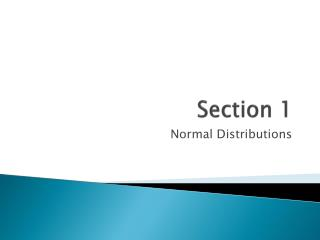# Section 1 - PowerPoint PPT PresentationDownload PresentationSection 1

Section 1Download Presentation## Section 1

- - - - - - - - - - - - - - - - - - - - - - - - - - - E N D - - - - - - - - - - - - - - - - - - - - - - - - - - -
##### Presentation Transcript

1. Section 1 Normal Distributions

2. Types of Distributions • Symmetric Distribution • Any normal distribution is symmetric Negatively Skewed (Left-skewed) distribution When a majority of the data values fall to the right of the mean Positively Skewed (Right-skewed) distribution when a majority of the data values fall to the left of the mean

3. Negatively Skewed • Positively Skewed Mean Med Mode Mean med mode Falls to the right of the data (Left-skewed) Falls to the left of the data (Right skewed)

4. Normal Distributions • The mean, median, and mode are all approximately the same. • Bell-shaped curve • Mean, median, and mode are equal and at the center • Only has one mode • Symmetric • NEVER touches the x-axis • Area under the curve is 100% or 1.00 • Fits the Empirical (Normal) Rule

5. Look at Figure 6-5 on page 304. • Notice the values are symmetric… this is true for ALL normal distributions.

6. The Standard Normal Distribution • A normal distribution with a mean of 0 and a standard deviation of 1. • We use this to approximate the area under the curve for any given Normal distribution • Use z-scores to do this (remember these from chapter 3…) • We will also use Table E to help us with the math.

7. Finding Areas Under the Standard Normal Distribution Curve • There are three different possibilities for where the area under the curve that you are looking for is located: • 1. To the left of the z-score • 2. To the right of the z-score • 3. Between any two z-scores

8. Area to the Left of any z-score • Look up the z-score using Table E (p. 784) • That is your answer • Ex. Find the area to the left of z = 2.06. • What does this mean?

9. Area to the Right of any z-score • Look up the z-score • SUBTRACT the area from 1 • Ex: Find the area to the right of z = -1.19

10. Between any two z-scores • Look up both z- scores • SUBTRACT the corresponding area (values) • Ex: Find the area between z = +1.68 and z = -1.37

11. You try these…. • 1. Area to the left of z = +.6 • 2. Area to the right of z = +1.04 • 3. Area to the right of z = -2.74 • 4. Area to the left of z = -0.32 • 5. Area between z = +0.13 and z = +1.40 • 6. Area between z = +1.03 and z = -0.23

12. Normal Distribution Curve as a Probability Distribution Curve • Remember that a Normal distribution is a continuous distribution • We can use z-scores to find the probability of choosing any z-value at random • A special notation is used: • If we are finding the probability of any z value between a and b, it is written as: P(a<z<b)

13. Examples • Find each of the probabilities • 1. P(0 < z < 2.32) • 2. P(z<1.54) • 3. P(z>1.91) • 4. P(1.21 < z < 2.34)

14. Working backwards…. • What is the z-value such that the area under the standard normal distribution curve is .2389?

15. The rules • 1. If it is on the negative side of zero, simply find the value. • 2. If it is on the positive side of zero, subtract the value from 1 and find the difference value. • 3. If it is between 0 and a number larger than zero, add .5 and then find the value. • 4. If it is between 0 and a number smaller than zero, subtract .5 and then find the value.

16. Working backwards…. • What is the z-value such that the area under the standard normal distribution curve between 0 and z is .2389?

17. Find the z value to the left of the mean such that 23.58% of the area lies to the right of it.

18. Classwork/Homework • P. 311